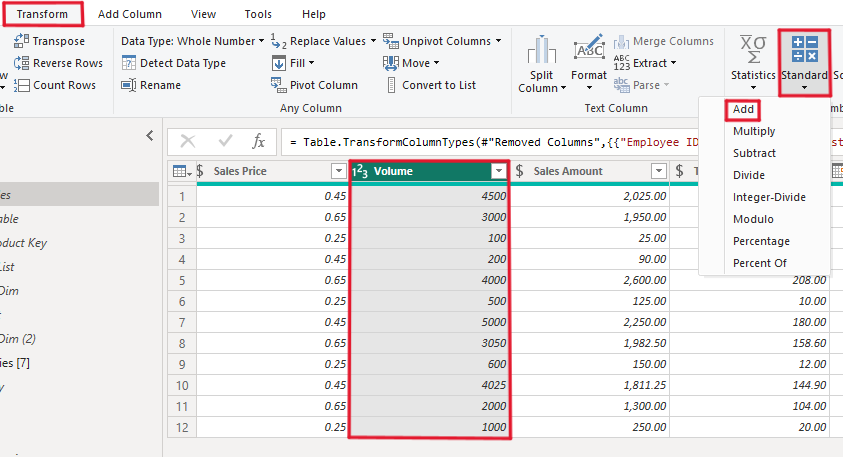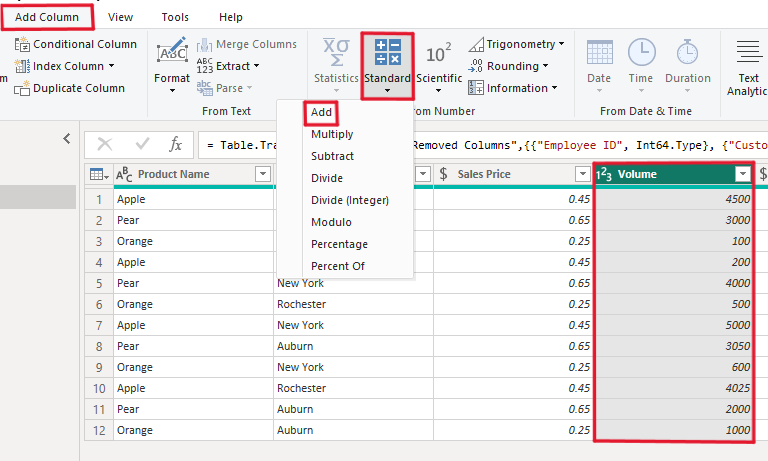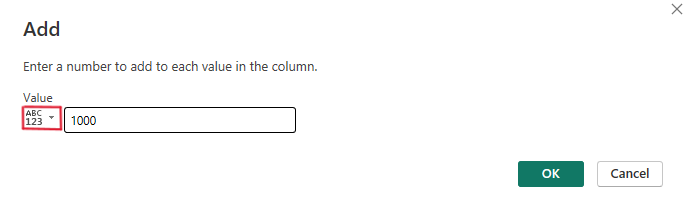Email : info@epmstrategy.com | Call Us Now : 479-321-3977
:: Home :: Sitemap ::

# Power BI – Arithmetic in Power Query

BY: David Rohlfs

Introduction

For many people that are used to Power BI, we tend to see a lot of functions and know what most of them do, but we only use a couple of them. One set of these functions that I tend to rarely use are the Arithmetic based functions in Power Query. These functions are basic and tend to be lightly used, but they can become very helpful if you need them.

In this blog we will go over the basics of using some of the arithmetic based functions in Power Query. This blog will not be going into depth on any specific function but is meant to be an overview of the entire group.

When to use it

If you don’t already know, what I mean by the arithmetic functions are the Number Column functions under the Transform ribbon and the From Number functions under the Add Column ribbon.There are six subgroups in the arithmetic group:

• Statistics
• Standard
• Scientific
• Trigonometry
• Rounding
• Information

Each of these groups has their own functions that pertain to their name. Here is the entire list of each subgroup and their functions:

Statistics:

• Sum
• Minimum
• Maximum
• Median
• Average
• Standard Deviation
• Count Values
• Count Distinct Values

Standard:

• Multiply
• Subtract
• Divide
• Integer-Divide
• Modulo
• Percentage
• Percent Of

Scientific:

• Absolute Value
• Power
• Square
• Cube
• Power…
• Square Root
• Exponent
• Logarithm
• Base 10
• Natural
• Factorial

Trigonometry:

• Sine
• Cosine
• Tangent
• Arcsine
• Arccosine
• Arctangent

Rounding:

• Round Up
• Round Down
• Round…

Information:

• Is Even
• Is Odd
• Sign

Common Problems

The most common problem that you will face is that your function may throw an error at you in a specific cell or cells. This will most likely happen when you have a value in your data that is not numerically based and is a text base value. Remember, Power Query only looks at the first 1000 rows to do its preview, so if rows 1001 or after has a text value, you will get an error for that row. An easy way to check if this is the case is to do a group by or sort.

Walkthrough

Because there are so many functions covered by the arithmetic groups in Power Query, we are not going to do a full walkthrough on all of them. Instead, I will be focusing on the addition function and show you the differences between using the Number Column and the Number From groups.

Here is the example of the Number Column (Transform Ribbon):

For these examples we will be using the Volume Column. My goal is to add 1,000 to each of the values in this column.With the volume column selected, I am going to go to the Transform ribbon, Standard, and select Add.Now I get a pop-out that is asking me the amount that I want to add to each of my values. In here I am just going to write in 1000 and click OK.Now you can see that in my original volume column I have added 1,000 to each of my values.Here is the example of the Number From (Add Column):

Like the Number Column walkthrough, my goal is to add 1,000 to each of my volume values. To do this I need to first have my volume column selected and go to the Add Column Ribbon, Standard, then Add.This will show a similar pop-out to the From Column process, but now we are able to add in values from a different column. So, if we wanted to, we could aggregate two columns together. But for this example, we are just going to type in 1000 then click OK.Now we should get a new column on the far right of our table that has the additional values. I have moved the Volume column so that you can easily see the before and after of this process.Conclusion

The basics of the arithmetic functions are very simple if you already know the math behind the functions itself. Hopefully you get to use the functions more as you work with Power Query and if you enjoyed this blog, I suggest reading through some of EPM Strategy’s other blogs related to Power Query linked below.

Power BI and Group BY

Power BI Column From Examples

Power BI: Append and Merge

M Code Basics

Count vs. Count Distinct

Replace Values Power Query

M Code If Statement